# General relativity

General relativity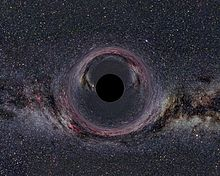A simulated black hole of ten solar masses as seen from a distance of 600 kilometers with the Milky Way in the background.

General relativity or the general theory of relativity is the geometric theory of gravitation published by Albert Einstein in 1916. It is a widely accepted description of gravitation in modern physics. General relativity generalises special relativity and Newton's law of universal gravitation, providing a unified description of gravity as a geometric property of space and time, or spacetime. In particular, the curvature of spacetime is directly related to the four-momentum (mass-energy and linear momentum) of whatever matter and radiation are present. The relation is specified by the Einstein field equations, a system of partial differential equations.

Some predictions of general relativity differ significantly from those of classical physics, especially concerning the passage of time, the geometry of space, the motion of bodies in free fall, and the propagation of light. Examples of such differences include gravitational time dilation, gravitational lensing, the gravitational redshift of light, and the gravitational time delay. General relativity's predictions have been confirmed in all observations and experiments to date. Although general relativity is not the only relativistic theory of gravity, it is the simplest theory that is consistent with experimental data. However, unanswered questions remain, the most fundamental being how general relativity can be reconciled with the laws of quantum physics to produce a complete and self-consistent theory of quantum gravity.

Einstein's theory has important astrophysical implications. For example, it implies the existence of black holes—regions of space in which space and time are distorted in such a way that nothing, not even light, can escape—as an end-state for massive stars. There is ample evidence that such stellar black holes as well as more massive varieties of black hole are responsible for the intense radiation emitted by certain types of astronomical objects such as active galactic nuclei or microquasars. The bending of light by gravity can lead to the phenomenon of gravitational lensing, where multiple images of the same distant astronomical object are visible in the sky. General relativity also predicts the existence of gravitational waves, which have since been measured indirectly; a direct measurement is the aim of projects such as LIGO and NASA/ESA Laser Interferometer Space Antenna. In addition, general relativity is the basis of current cosmological models of a consistently expanding universe.

## History

Soon after publishing the special theory of relativity in 1905, Einstein started thinking about how to incorporate gravity into his new relativistic framework. In 1907, beginning with a simple thought experiment involving an observer in free fall, he embarked on what would be an eight-year search for a relativistic theory of gravity. After numerous detours and false starts, his work culminated in the November, 1915 presentation to the Prussian Academy of Science of what are now known as the Einstein field equations. These equations specify how the geometry of space and time is influenced by whatever matter is present, and form the core of Einstein's general theory of relativity.

The Einstein field equations are nonlinear and very difficult to solve. Einstein used approximation methods in working out initial predictions of the theory. But as early as 1916, the astrophysicist Karl Schwarzschild found the first non-trivial exact solution to the Einstein field equations, the so-called Schwarzschild metric. This solution laid the groundwork for the description of the final stages of gravitational collapse, and the objects known today as black holes. In the same year, the first steps towards generalizing Schwarzschild's solution to electrically charged objects were taken, which eventually resulted in the Reissner-Nordström solution, now associated with electrically charged black holes. In 1917, Einstein applied his theory to the universe as a whole, initiating the field of relativistic cosmology. In line with contemporary thinking, he assumed a static universe, adding a new parameter to his original field equations—the cosmological constant—to reproduce that "observation". By 1929, however, the work of Hubble and others had shown that our universe is expanding. This is readily described by the expanding cosmological solutions found by Friedmann in 1922, which do not require a cosmological constant. Lemaître used these solutions to formulate the earliest version of the big bang models, in which our universe has evolved from an extremely hot and dense earlier state. Einstein later declared the cosmological constant the biggest blunder of his life.

During that period, general relativity remained something of a curiosity among physical theories. It was clearly superior to Newtonian gravity, being consistent with special relativity and accounting for several effects unexplained by the Newtonian theory. Einstein himself had shown in 1915 how his theory explained the anomalous perihelion advance of the planet Mercury without any arbitrary parameters ("fudge factors"). Similarly, a 1919 expedition led by Eddington confirmed general relativity's prediction for the deflection of starlight by the Sun during the total solar eclipse of May 29, 1919, making Einstein instantly famous. Yet the theory entered the mainstream of theoretical physics and astrophysics only with the developments between approximately 1960 and 1975, now known as the Golden age of general relativity. Physicists began to understand the concept of a black hole, and to identify these objects' astrophysical manifestation as quasars. Ever more precise solar system tests confirmed the theory's predictive power, and relativistic cosmology, too, became amenable to direct observational tests.

## From classical mechanics to general relativity

General relativity is best understood by examining its similarities with and departures from classical physics. The first step is the realization that classical mechanics and Newton's law of gravity admit of a geometric description. The combination of this description with the laws of special relativity results in a heuristic derivation of general relativity.

### Geometry of Newtonian gravity

At the base of classical mechanics is the notion that a body's motion can be described as a combination of free (or inertial) motion, and deviations from this free motion. Such deviations are caused by external forces acting on a body in accordance with Newton's second law of motion, which states that the net force acting on a body is equal to that body's (inertial) mass multiplied by its acceleration. The preferred inertial motions are related to the geometry of space and time: in the standard reference frames of classical mechanics, objects in free motion move along straight lines at constant speed. In modern parlance, their paths are geodesics, straight world lines in curved spacetime.According to general relativity, a ball will fall the same way in an accelerating rocket (left) as it does in a gravitational field, such as on Earth (right).

Conversely, one might expect that inertial motions, once identified by observing the actual motions of bodies and making allowances for the external forces (such as electromagnetism or friction), can be used to define the geometry of space, as well as a time coordinate. However, there is an ambiguity once gravity comes into play. According to Newton's law of gravity, and independently verified by experiments such as that of Eötvös and its successors (see Eötvös experiment), there is a universality of free fall (also known as the weak equivalence principle, or the universal equality of inertial and passive-gravitational mass): the trajectory of a test body in free fall depends only on its position and initial speed, but not on any of its material properties. A simplified version of this is embodied in Einstein's elevator experiment, illustrated in the figure on the right: for an observer in a small enclosed room, it is impossible to decide, by mapping the trajectory of bodies such as a dropped ball, whether the room is at rest in a gravitational field, or in free space aboard an accelerating rocket generating a force equal to gravity.

Given the universality of free fall, there is no observable distinction between inertial motion and motion under the influence of the gravitational force. This suggests the definition of a new class of inertial motion, namely that of objects in free fall under the influence of gravity. This new class of preferred motions, too, defines a geometry of space and time—in mathematical terms, it is the geodesic motion associated with a specific connection which depends on the gradient of the gravitational potential. Space, in this construction, still has the ordinary Euclidean geometry. However, spacetime as a whole is more complicated. As can be shown using simple thought experiments following the free-fall trajectories of different test particles, the result of transporting spacetime vectors that can denote a particle's velocity (time-like vectors) will vary with the particle's trajectory; mathematically speaking, the Newtonian connection is not integrable. From this, one can deduce that spacetime is curved. The result is a geometric formulation of Newtonian gravity using only covariant concepts, i.e. a description which is valid in any desired coordinate system. In this geometric description, tidal effects—the relative acceleration of bodies in free fall—are related to the derivative of the connection, showing how the modified geometry is caused by the presence of mass.

### Relativistic generalization

As intriguing as geometric Newtonian gravity may be, its basis, classical mechanics, is merely a limiting case of (special) relativistic mechanics. In the language of symmetry: where gravity can be neglected, physics is Lorentz invariant as in special relativity rather than Galilei invariant as in classical mechanics. (The defining symmetry of special relativity is the Poincaré group which also includes translations and rotations.) The differences between the two become significant when we are dealing with speeds approaching the speed of light, and with high-energy phenomena.

With Lorentz symmetry, additional structures come into play. They are defined by the set of light cones (see the image on the left). The light-cones define a causal structure: for each event A, there is a set of events that can, in principle, either influence or be influenced by A via signals or interactions that do not need to travel faster than light (such as event B in the image), and a set of events for which such an influence is impossible (such as event C in the image). These sets are observer-independent. In conjunction with the world-lines of freely falling particles, the light-cones can be used to reconstruct the space–time's semi-Riemannian metric, at least up to a positive scalar factor. In mathematical terms, this defines a conformal structure.

Special relativity is defined in the absence of gravity, so for practical applications, it is a suitable model whenever gravity can be neglected. Bringing gravity into play, and assuming the universality of free fall, an analogous reasoning as in the previous section applies: there are no global inertial frames. Instead there are approximate inertial frames moving alongside freely falling particles. Translated into the language of spacetime: the straight time-like lines that define a gravity-free inertial frame are deformed to lines that are curved relative to each other, suggesting that the inclusion of gravity necessitates a change in spacetime geometry.

A priori, it is not clear whether the new local frames in free fall coincide with the reference frames in which the laws of special relativity hold—that theory is based on the propagation of light, and thus on electromagnetism, which could have a different set of preferred frames. But using different assumptions about the special-relativistic frames (such as their being earth-fixed, or in free fall), one can derive different predictions for the gravitational redshift, that is, the way in which the frequency of light shifts as the light propagates through a gravitational field (cf. below). The actual measurements show that free-falling frames are the ones in which light propagates as it does in special relativity. The generalization of this statement, namely that the laws of special relativity hold to good approximation in freely falling (and non-rotating) reference frames, is known as the Einstein equivalence principle, a crucial guiding principle for generalizing special-relativistic physics to include gravity.

The same experimental data shows that time as measured by clocks in a gravitational field—proper time, to give the technical term—does not follow the rules of special relativity. In the language of spacetime geometry, it is not measured by the Minkowski metric. As in the Newtonian case, this is suggestive of a more general geometry. At small scales, all reference frames that are in free fall are equivalent, and approximately Minkowskian. Consequently, we are now dealing with a curved generalization of Minkowski space. The metric tensor that defines the geometry—in particular, how lengths and angles are measured—is not the Minkowski metric of special relativity, it is a generalization known as a semi- or pseudo-Riemannian metric. Furthermore, each Riemannian metric is naturally associated with one particular kind of connection, the Levi-Civita connection, and this is, in fact, the connection that satisfies the equivalence principle and makes space locally Minkowskian (that is, in suitable locally inertial coordinates, the metric is Minkowskian, and its first partial derivatives and the connection coefficients vanish).

### Einstein's equations

Having formulated the relativistic, geometric version of the effects of gravity, the question of gravity's source remains. In Newtonian gravity, the source is mass. In special relativity, mass turns out to be part of a more general quantity called the energy-momentum tensor, which includes both energy and momentum densities as well as stress (that is, pressure and shear). Using the equivalence principle, this tensor is readily generalized to curved space-time. Drawing further upon the analogy with geometric Newtonian gravity, it is natural to assume that the field equation for gravity relates this tensor and the Ricci tensor, which describes a particular class of tidal effects: the change in volume for a small cloud of test particles that are initially at rest, and then fall freely. In special relativity, conservation of energy-momentum corresponds to the statement that the energy-momentum tensor is divergence-free. This formula, too, is readily generalized to curved spacetime by replacing partial derivatives with their curved-manifold counterparts, covariant derivatives studied in differential geometry. With this additional condition—the covariant divergence of the energy-momentum tensor, and hence of whatever is on the other side of the equation, is zero— the simplest set of equations are what are called Einstein's (field) equations:$R_{ab} - {\textstyle 1 \over 2}R\,g_{ab} = {8 \pi G \over c^4} T_{ab}.\,$

On the left-hand side is the Einstein tensor, a specific divergence-free combination of the Ricci tensor Rab and the metric. In particular,$R=R_{cd}g^{cd}\,$

is the curvature scalar. The Ricci tensor itself is related to the more general Riemann curvature tensor as$\quad R_{ab}={R^d}_{adb}.\,$

On the right-hand side, Tab is the energy-momentum tensor. All tensors are written in abstract index notation. Matching the theory's prediction to observational results for planetary orbits (or, equivalently, assuring that the weak-gravity, low-speed limit is Newtonian mechanics), the proportionality constant can be fixed as κ = 8πG/c4, with G the gravitational constant and c the speed of light. When there is no matter present, so that the energy-momentum tensor vanishes, the result are the vacuum Einstein equations,$R_{ab}=0.\,$

There are alternatives to general relativity built upon the same premises, which include additional rules and/or constraints, leading to different field equations. Examples are Brans-Dicke theory, teleparallelism, and Einstein-Cartan theory.

## Definition and basic applications

The derivation outlined in the previous section contains all the information needed to define general relativity, describe its key properties, and address a question of crucial importance in physics, namely how the theory can be used for model-building.

### Definition and basic properties

General relativity is a metric theory of gravitation. At its core are Einstein's equations, which describe the relation between the geometry of a four-dimensional, pseudo-Riemannian manifold representing spacetime, and the energy-momentum contained in that spacetime. Phenomena that in classical mechanics are ascribed to the action of the force of gravity (such as free-fall, orbital motion, and spacecraft trajectories), correspond to inertial motion within a curved geometry of spacetime in general relativity; there is no gravitational force deflecting objects from their natural, straight paths. Instead, gravity corresponds to changes in the properties of space and time, which in turn changes the straightest-possible paths that objects will naturally follow. The curvature is, in turn, caused by the energy-momentum of matter. Paraphrasing the relativist John Archibald Wheeler, spacetime tells matter how to move; matter tells spacetime how to curve.

While general relativity replaces the scalar gravitational potential of classical physics by a symmetric rank-two tensor, the latter reduces to the former in certain limiting cases. For weak gravitational fields and slow speed relative to the speed of light, the theory's predictions converge on those of Newton's law of universal gravitation.

As it is constructed using tensors, general relativity exhibits general covariance: its laws—and further laws formulated within the general relativistic framework—take on the same form in all coordinate systems. Furthermore, the theory does not contain any invariant geometric background structures, i.e. it is background independent. It thus satisfies a more stringent general principle of relativity, namely that the laws of physics are the same for all observers. Locally, as expressed in the equivalence principle, spacetime is Minkowskian, and the laws of physics exhibit local Lorentz invariance.

### Model-building

The core concept of general-relativistic model-building is that of a solution of Einstein's equations. Given both Einstein's equations and suitable equations for the properties of matter, such a solution consists of a specific semi-Riemannian manifold (usually defined by giving the metric in specific coordinates), and specific matter fields defined on that manifold. Matter and geometry must satisfy Einstein's equations, so in particular, the matter's energy-momentum tensor must be divergence-free. The matter must, of course, also satisfy whatever additional equations were imposed on its properties. In short, such a solution is a model universe that satisfies the laws of general relativity, and possibly additional laws governing whatever matter might be present.

Einstein's equations are nonlinear partial differential equations and, as such, difficult to solve exactly. Nevertheless, a number of exact solutions are known, although only a few have direct physical applications. The best-known exact solutions, and also those most interesting from a physics point of view, are the Schwarzschild solution, the Reissner-Nordström solution and the Kerr metric, each corresponding to a certain type of black hole in an otherwise empty universe, and the Friedmann-Lemaître-Robertson-Walker and de Sitter universes, each describing an expanding cosmos. Exact solutions of great theoretical interest include the Gödel universe (which opens up the intriguing possibility of time travel in curved spacetimes), the Taub-NUT solution (a model universe that is homogeneous, but anisotropic), and Anti-de Sitter space (which has recently come to prominence in the context of what is called the Maldacena conjecture).

Given the difficulty of finding exact solutions, Einstein's field equations are also solved frequently by numerical integration on a computer, or by considering small perturbations of exact solutions. In the field of numerical relativity, powerful computers are employed to simulate the geometry of spacetime and to solve Einstein's equations for interesting situations such as two colliding black holes. In principle, such methods may be applied to any system, given sufficient computer resources, and may address fundamental questions such as naked singularities. Approximate solutions may also be found by perturbation theories such as linearized gravity and its generalization, the post-Newtonian expansion, both of which were developed by Einstein. The latter provides a systematic approach to solving for the geometry of a spacetime that contains a distribution of matter that moves slowly compared with the speed of light. The expansion involves a series of terms; the first terms represent Newtonian gravity, whereas the later terms represent ever smaller corrections to Newton's theory due to general relativity. An extension of this expansion is the parametrized post-Newtonian (PPN) formalism, which allows quantitative comparisons between the predictions of general relativity and alternative theories.

## Consequences of Einstein's theory

General relativity has a number of physical consequences. Some follow directly from the theory's axioms, whereas others have become clear only in the course of the ninety years of research that followed Einstein's initial publication.

### Gravitational time dilation and frequency shift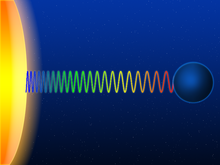Schematic representation of the gravitational redshift of a light wave escaping from the surface of a massive body

Assuming that the equivalence principle holds, gravity influences the passage of time. Light sent down into a gravity well is blueshifted, whereas light sent in the opposite direction (i.e., climbing out of the gravity well) is redshifted; collectively, these two effects are known as the gravitational frequency shift. More generally, processes close to a massive body run more slowly when compared with processes taking place farther away; this effect is known as gravitational time dilation.

Gravitational redshift has been measured in the laboratory and using astronomical observations. Gravitational time dilation in the Earth's gravitational field has been measured numerous times using atomic clocks, while ongoing validation is provided as a side effect of the operation of the Global Positioning System (GPS). Tests in stronger gravitational fields are provided by the observation of binary pulsars. All results are in agreement with general relativity. However, at the current level of accuracy, these observations cannot distinguish between general relativity and other theories in which the equivalence principle is valid.

### Light deflection and gravitational time delay

General relativity predicts that the path of light is bent in a gravitational field; light passing a massive body is deflected towards that body. This effect has been confirmed by observing the light of stars or distant quasars being deflected as it passes the Sun.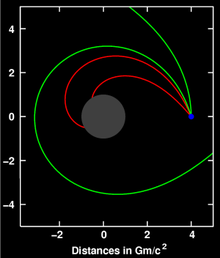Deflection of light (sent out from the location shown in blue) near a compact body (shown in gray)

This and related predictions follow from the fact that light follows what is called a light-like or null geodesic—a generalization of the straight lines along which light travels in classical physics. Such geodesics are the generalization of the invariance of lightspeed in special relativity. As one examines suitable model spacetimes (either the exterior Schwarzschild solution or, for more than a single mass, the post-Newtonian expansion), several effects of gravity on light propagation emerge. Although the bending of light can also be derived by extending the universality of free fall to light, the angle of deflection resulting from such calculations is only half the value given by general relativity.

Closely related to light deflection is the gravitational time delay (or Shapiro effect), the phenomenon that light signals take longer to move through a gravitational field than they would in the absence of that field. There have been numerous successful tests of this prediction. In the parameterized post-Newtonian formalism (PPN), measurements of both the deflection of light and the gravitational time delay determine a parameter called γ, which encodes the influence of gravity on the geometry of space.

### Gravitational waves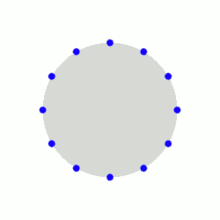Ring of test particles influenced by gravitational wave

One of several analogies between weak-field gravity and electromagnetism is that, analogous to electromagnetic waves, there are gravitational waves: ripples in the metric of spacetime that propagate at the speed of light. The simplest type of such a wave can be visualized by its action on a ring of freely floating particles. A sine wave propagating through such a ring towards the reader distorts the ring in a characteristic, rhythmic fashion (animated image to the right). Since Einstein's equations are non-linear, arbitrarily strong gravitational waves do not obey linear superposition, making their description difficult. However, for weak fields, a linear approximation can be made. Such linearized gravitational waves are sufficiently accurate to describe the exceedingly weak waves that are expected to arrive here on Earth from far-off cosmic events, which typically result in relative distances increasing and decreasing by 10 − 21 or less. Data-analysis methods routinely make use of the fact that these linearized waves can be Fourier decomposed.

Some exact solutions describe gravitational waves without any approximation, e.g., a wave train traveling through empty space or so-called Gowdy universes, varieties of an expanding cosmos filled with gravitational waves. But for gravitational waves produced in astrophysically relevant situations, such as the merger of two black holes, numerical methods are presently the only way to construct appropriate models.

### Orbital effects and the relativity of direction

General relativity differs from classical mechanics in a number of predictions concerning orbiting bodies. It predicts an overall rotation (precession) of planetary orbits, as well as orbital decay caused by the emission of gravitational waves and effects related to the relativity of direction.

#### Precession of apsides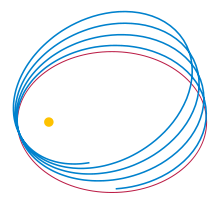Newtonian (red) vs. Einsteinian orbit (blue) of a lone planet orbiting a star

In general relativity, the apsides of any orbit (the point of the orbiting body's closest approach to the system's center of mass) will precess—the orbit is not an ellipse, but akin to an ellipse that rotates on its focus, resulting in a rose curve-like shape (see image). Einstein first derived this result by using an approximate metric representing the Newtonian limit and treating the orbiting body as a test particle. For him, the fact that his theory gave a straightforward explanation of the anomalous perihelion shift of the planet Mercury, discovered earlier by Urbain Le Verrier in 1859, was important evidence that he had at last identified the correct form of the gravitational field equations.

The effect can also be derived by using either the exact Schwarzschild metric (describing spacetime around a spherical mass) or the much more general post-Newtonian formalism. It is due to the influence of gravity on the geometry of space and to the contribution of self-energy to a body's gravity (encoded in the nonlinearity of Einstein's equations). Relativistic precession has been observed for all planets that allow for accurate precession measurements (Mercury, Venus and the Earth), as well as in binary pulsar systems, where it is larger by five orders of magnitude.

#### Orbital decayOrbital decay for PSR1913+16: time shift in seconds, tracked over three decades.

According to general relativity, a binary system will emit gravitational waves, thereby losing energy. Due to this loss, the distance between the two orbiting bodies decreases, and so does their orbital period. Within the solar system or for ordinary double stars, the effect is too small to be observable. This is not the case for a close binary pulsar, a system of two orbiting neutron stars, one of which is a pulsar: from the pulsar, observers on Earth receive a regular series of radio pulses that can serve as a highly accurate clock, which allows precise measurements of the orbital period. Since the neutron stars are very compact, significant amounts of energy are emitted in the form of gravitational radiation.

The first observation of a decrease in orbital period due to the emission of gravitational waves was made by Hulse and Taylor, using the binary pulsar PSR1913+16 they had discovered in 1974. This was the first detection of gravitational waves, albeit indirect, for which they were awarded the 1993 Nobel Prize in physics. Since then, several other binary pulsars have been found, in particular the double pulsar PSR J0737-3039, in which both stars are pulsars.

#### Geodetic precession and frame-dragging

Several relativistic effects are directly related to the relativity of direction. One is geodetic precession: the axis direction of a gyroscope in free fall in curved spacetime will change when compared, for instance, with the direction of light received from distant stars—even though such a gyroscope represents the way of keeping a direction as stable as possible ("parallel transport"). For the Moon-Earth-system, this effect has been measured with the help of lunar laser ranging. More recently, it has been measured for test masses aboard the satellite Gravity Probe B to a precision of better than 1%.

Near a rotating mass, there are so-called gravitomagnetic or frame-dragging effects. A distant observer will determine that objects close to the mass get "dragged around". This is most extreme for rotating black holes where, for any object entering a zone known as the ergosphere, rotation is inevitable. Such effects can again be tested through their influence on the orientation of gyroscopes in free fall. Somewhat controversial tests have been performed using the LAGEOS satellites, confirming the relativistic prediction. Also the Mars Global Surveyor probe around Mars has been used.

A precision measurement is the main aim of the Gravity Probe B mission. The geodetic effect was confirmed to better than 0.5% accuracy.

## Astrophysical applications

### Gravitational lensingEinstein cross: four images of the same astronomical object, produced by a gravitational lens

The deflection of light by gravity is responsible for a new class of astronomical phenomena. If a massive object is situated between the astronomer and a distant target object with appropriate mass and relative distances, the astronomer will see multiple distorted images of the target. Such effects are known as gravitational lensing. Depending on the configuration, scale, and mass distribution, there can be two or more images, a bright ring known as an Einstein ring, or partial rings called arcs. The earliest example was discovered in 1979; since then, more than a hundred gravitational lenses have been observed. Even if the multiple images are too close to each other to be resolved, the effect can still be measured, e.g., as an overall brightening of the target object; a number of such "microlensing events" have been observed.

Gravitational lensing has developed into a tool of observational astronomy. It is used to detect the presence and distribution of dark matter, provide a "natural telescope" for observing distant galaxies, and to obtain an independent estimate of the Hubble constant. Statistical evaluations of lensing data provide valuable insight into the structural evolution of galaxies.

### Gravitational wave astronomy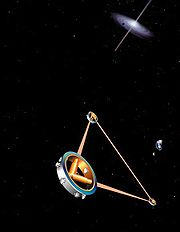Artist's impression of the space-borne gravitational wave detector LISA

Observations of binary pulsars provide strong indirect evidence for the existence of gravitational waves (see Orbital decay, above). However, gravitational waves reaching us from the depths of the cosmos have not been detected directly, which is a major goal of current relativity-related research. Several land-based gravitational wave detectors are currently in operation, most notably the interferometric detectors GEO 600, LIGO (three detectors), TAMA 300 and VIRGO. A joint US-European space-based detector, LISA, is currently under development, with a precursor mission (LISA Pathfinder) due for launch in 2012.

Observations of gravitational waves promise to complement observations in the electromagnetic spectrum. They are expected to yield information about black holes and other dense objects such as neutron stars and white dwarfs, about certain kinds of supernova implosions, and about processes in the very early universe, including the signature of certain types of hypothetical cosmic string.

### Black holes and other compact objects

Whenever the ratio of an object's mass to its radius becomes sufficiently large, general relativity predicts the formation of a black hole, a region of space from which nothing, not even light, can escape. In the currently accepted models of stellar evolution, neutron stars of around 1.4 solar masses, and stellar black holes with a few to a few dozen solar masses, are thought to be the final state for the evolution of massive stars. Usually a galaxy has one supermassive black hole with a few million to a few billion solar masses in its center, and its presence is thought to have played an important role in the formation of the galaxy and larger cosmic structures.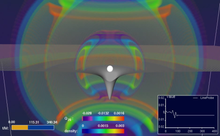Simulation based on the equations of general relativity: a star collapsing to form a black hole while emitting gravitational waves

Astronomically, the most important property of compact objects is that they provide a supremely efficient mechanism for converting gravitational energy into electromagnetic radiation. Accretion, the falling of dust or gaseous matter onto stellar or supermassive black holes, is thought to be responsible for some spectacularly luminous astronomical objects, notably diverse kinds of active galactic nuclei on galactic scales and stellar-size objects such as microquasars. In particular, accretion can lead to relativistic jets, focused beams of highly energetic particles that are being flung into space at almost light speed. General relativity plays a central role in modelling all these phenomena, and observations provide strong evidence for the existence of black holes with the properties predicted by the theory.

Black holes are also sought-after targets in the search for gravitational waves (cf. Gravitational waves, above). Merging black hole binaries should lead to some of the strongest gravitational wave signals reaching detectors here on Earth, and the phase directly before the merger ("chirp") could be used as a "standard candle" to deduce the distance to the merger events–and hence serve as a probe of cosmic expansion at large distances. The gravitational waves produced as a stellar black hole plunges into a supermassive one should provide direct information about supermassive black hole's geometry.

### Cosmology

The current models of cosmology are based on Einstein's field equations, which include the cosmological constant Λ since it has important influence on the large-scale dynamics of the cosmos,$R_{ab} - {\textstyle 1 \over 2}R\,g_{ab} + \Lambda\ g_{ab} = \kappa\, T_{ab}$

where gab is the spacetime metric. Isotropic and homogeneous solutions of these enhanced equations, the Friedmann-Lemaître-Robertson-Walker solutions, allow physicists to model a universe that has evolved over the past 14 billion years from a hot, early Big Bang phase. Once a small number of parameters (for example the universe's mean matter density) have been fixed by astronomical observation, further observational data can be used to put the models to the test. Predictions, all successful, include the initial abundance of chemical elements formed in a period of primordial nucleosynthesis, the large-scale structure of the universe, and the existence and properties of a "thermal echo" from the early cosmos, the cosmic background radiation.

Astronomical observations of the cosmological expansion rate allow the total amount of matter in the universe to be estimated, although the nature of that matter remains mysterious in part. About 90% of all matter appears to be so-called dark matter, which has mass (or, equivalently, gravitational influence), but does not interact electromagnetically and, hence, cannot be observed directly. There is no generally accepted description of this new kind of matter, within the framework of known particle physics or otherwise. Observational evidence from redshift surveys of distant supernovae and measurements of the cosmic background radiation also show that the evolution of our universe is significantly influenced by a cosmological constant resulting in an acceleration of cosmic expansion or, equivalently, by a form of energy with an unusual equation of state, known as dark energy, the nature of which remains unclear.

A so-called inflationary phase, an additional phase of strongly accelerated expansion at cosmic times of around 10 − 33 seconds, was hypothesized in 1980 to account for several puzzling observations that were unexplained by classical cosmological models, such as the nearly perfect homogeneity of the cosmic background radiation. Recent measurements of the cosmic background radiation have resulted in the first evidence for this scenario. However, there is a bewildering variety of possible inflationary scenarios, which cannot be restricted by current observations. An even larger question is the physics of the earliest universe, prior to the inflationary phase and close to where the classical models predict the big bang singularity. An authoritative answer would require a complete theory of quantum gravity, which has not yet been developed (cf. the section on quantum gravity, below).

### Causal structure and global geometry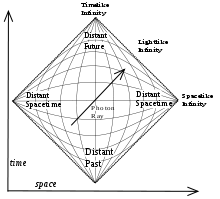Penrose diagram of an infinite Minkowski universe

In general relativity, no material body can catch up with or overtake a light pulse. No influence from an event A can reach any other location X before light sent out at A to X. In consequence, an exploration of all light worldlines (null geodesics) yields key information about the spacetime's causal structure. This structure can be displayed using Penrose-Carter diagrams in which infinitely large regions of space and infinite time intervals are shrunk ("compactified") so as to fit onto a finite map, while light still travels along diagonals as in standard spacetime diagrams.

Aware of the importance of causal structure, Roger Penrose and others developed what is known as global geometry. In global geometry, the object of study is not one particular solution (or family of solutions) to Einstein's equations. Rather, relations that hold true for all geodesics, such as the Raychaudhuri equation, and additional non-specific assumptions about the nature of matter (usually in the form of so-called energy conditions) are used to derive general results.

### Horizons

Using global geometry, some spacetimes can be shown to contain boundaries called horizons, which demarcate one region from the rest of spacetime. The best-known examples are black holes: if mass is compressed into a sufficiently compact region of space (as specified in the hoop conjecture, the relevant length scale is the Schwarzschild radius), no light from inside can escape to the outside. Since no object can overtake a light pulse, all interior matter is imprisoned as well. Passage from the exterior to the interior is still possible, showing that the boundary, the black hole's horizon, is not a physical barrier.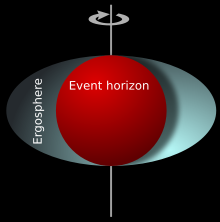The ergosphere of a rotating black hole, which plays a key role when it comes to extracting energy from such a black hole

Early studies of black holes relied on explicit solutions of Einstein's equations, notably the spherically symmetric Schwarzschild solution (used to describe a static black hole) and the axisymmetric Kerr solution (used to describe a rotating, stationary black hole, and introducing interesting features such as the ergosphere). Using global geometry, later studies have revealed more general properties of black holes. In the long run, they are rather simple objects characterized by eleven parameters specifying energy, linear momentum, angular momentum, location at a specified time and electric charge. This is stated by the black hole uniqueness theorems: "black holes have no hair", that is, no distinguishing marks like the hairstyles of humans. Irrespective of the complexity of a gravitating object collapsing to form a black hole, the object that results (having emitted gravitational waves) is very simple.

Even more remarkably, there is a general set of laws known as black hole mechanics, which is analogous to the laws of thermodynamics. For instance, by the second law of black hole mechanics, the area of the event horizon of a general black hole will never decrease with time, analogous to the entropy of a thermodynamic system. This limits the energy that can be extracted by classical means from a rotating black hole (e.g. by the Penrose process). There is strong evidence that the laws of black hole mechanics are, in fact, a subset of the laws of thermodynamics, and that the black hole area is proportional to its entropy. This leads to a modification of the original laws of black hole mechanics: for instance, as the second law of black hole mechanics becomes part of the second law of thermodynamics, it is possible for black hole area to decrease—as long as other processes ensure that, overall, entropy increases. As thermodynamical objects with non-zero temperature, black holes should emit thermal radiation. Semi-classical calculations indicate that indeed they do, with the surface gravity playing the role of temperature in Planck's law. This radiation is known as Hawking radiation (cf. the quantum theory section, below).

There are other types of horizons. In an expanding universe, an observer may find that some regions of the past cannot be observed ("particle horizon"), and some regions of the future cannot be influenced (event horizon). Even in flat Minkowski space, when described by an accelerated observer (Rindler space), there will be horizons associated with a semi-classical radiation known as Unruh radiation.

### Singularities

Another general—and quite disturbing—feature of general relativity is the appearance of spacetime boundaries known as singularities. Spacetime can be explored by following up on timelike and lightlike geodesics—all possible ways that light and particles in free fall can travel. But some solutions of Einstein's equations have "ragged edges"—regions known as spacetime singularities, where the paths of light and falling particles come to an abrupt end, and geometry becomes ill-defined. In the more interesting cases, these are "curvature singularities", where geometrical quantities characterizing spacetime curvature, such as the Ricci scalar, take on infinite values. Well-known examples of spacetimes with future singularities—where worldlines end—are the Schwarzschild solution, which describes a singularity inside an eternal static black hole, or the Kerr solution with its ring-shaped singularity inside an eternal rotating black hole. The Friedmann-Lemaître-Robertson-Walker solutions and other spacetimes describing universes have past singularities on which worldlines begin, namely big bang singularities, and some have future singularities (big crunch) as well.

Given that these examples are all highly symmetric—and thus simplified—it is tempting to conclude that the occurrence of singularities is an artefact of idealization. The famous singularity theorems, proved using the methods of global geometry, say otherwise: singularities are a generic feature of general relativity, and unavoidable once the collapse of an object with realistic matter properties has proceeded beyond a certain stage and also at the beginning of a wide class of expanding universes. However, the theorems say little about the properties of singularities, and much of current research is devoted to characterizing these entities' generic structure (hypothesized e.g. by the so-called BKL conjecture). The cosmic censorship hypothesis states that all realistic future singularities (no perfect symmetries, matter with realistic properties) are safely hidden away behind a horizon, and thus invisible to all distant observers. While no formal proof yet exists, numerical simulations offer supporting evidence of its validity.

### Evolution equations

Each solution of Einstein's equation encompasses the whole history of a universe — it is not just some snapshot of how things are, but a whole, possibly matter-filled, spacetime. It describes the state of matter and geometry everywhere and at every moment in that particular universe. Due to its general covariance, Einstein's theory is not sufficient by itself to determine the time evolution of the metric tensor. It must be combined with a coordinate condition, which is analogous to gauge fixing in other field theories.

To understand Einstein's equations as partial differential equations, it is helpful to formulate them in a way that describes the evolution of the universe over time. This is done in so-called "3+1" formulations, where spacetime is split into three space dimensions and one time dimension. The best-known example is the ADM formalism. These decompositions show that the spacetime evolution equations of general relativity are well-behaved: solutions always exist, and are uniquely defined, once suitable initial conditions have been specified. Such formulations of Einstein's field equations are the basis of numerical relativity.

### Global and quasi-local quantities

The notion of evolution equations is intimately tied in with another aspect of general relativistic physics. In Einstein's theory, it turns out to be impossible to find a general definition for a seemingly simple property such as a system's total mass (or energy). The main reason is that the gravitational field—like any physical field—must be ascribed a certain energy, but that it proves to be fundamentally impossible to localize that energy.

Nevertheless, there are possibilities to define a system's total mass, either using a hypothetical "infinitely distant observer" (ADM mass) or suitable symmetries (Komar mass). If one excludes from the system's total mass the energy being carried away to infinity by gravitational waves, the result is the so-called Bondi mass at null infinity. Just as in classical physics, it can be shown that these masses are positive. Corresponding global definitions exist for momentum and angular momentum. There have also been a number of attempts to define quasi-local quantities, such as the mass of an isolated system formulated using only quantities defined within a finite region of space containing that system. The hope is to obtain a quantity useful for general statements about isolated systems, such as a more precise formulation of the hoop conjecture.

## Relationship with quantum theory

If general relativity is considered one of the two pillars of modern physics, quantum theory, the basis of understanding matter from elementary particles to solid state physics, is the other. However, it is still an open question as to how the concepts of quantum theory can be reconciled with those of general relativity.

### Quantum field theory in curved spacetime

Ordinary quantum field theories, which form the basis of modern elementary particle physics, are defined in flat Minkowski space, which is an excellent approximation when it comes to describing the behavior of microscopic particles in weak gravitational fields like those found on Earth. In order to describe situations in which gravity is strong enough to influence (quantum) matter, yet not strong enough to require quantization itself, physicists have formulated quantum field theories in curved spacetime. These theories rely on classical general relativity to describe a curved background spacetime, and define a generalized quantum field theory to describe the behavior of quantum matter within that spacetime. Using this formalism, it can be shown that black holes emit a blackbody spectrum of particles known as Hawking radiation, leading to the possibility that they evaporate over time. As briefly mentioned above, this radiation plays an important role for the thermodynamics of black holes.

### Quantum gravity

The demand for consistency between a quantum description of matter and a geometric description of spacetime, as well as the appearance of singularities (where curvature length scales become microscopic), indicate the need for a full theory of quantum gravity: for an adequate description of the interior of black holes, and of the very early universe, a theory is required in which gravity and the associated geometry of spacetime are described in the language of quantum physics. Despite major efforts, no complete and consistent theory of quantum gravity is currently known, even though a number of promising candidates exist.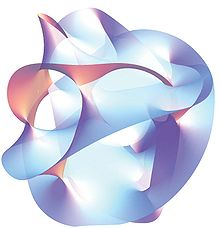Projection of a Calabi-Yau manifold, one of the ways of compactifying the extra dimensions posited by string theory

Attempts to generalize ordinary quantum field theories, used in elementary particle physics to describe fundamental interactions, so as to include gravity have led to serious problems. At low energies, this approach proves successful, in that it results in an acceptable effective (quantum) field theory of gravity. At very high energies, however, the result are models devoid of all predictive power ("non-renormalizability").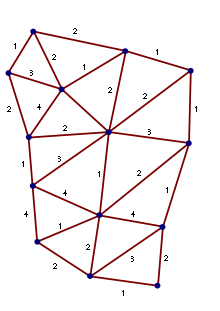Simple spin network of the type used in loop quantum gravity

One attempt to overcome these limitations is string theory, a quantum theory not of point particles, but of minute one-dimensional extended objects. The theory promises to be a unified description of all particles and interactions, including gravity; the price to pay is unusual features such as six extra dimensions of space in addition to the usual three. In what is called the second superstring revolution, it was conjectured that both string theory and a unification of general relativity and supersymmetry known as supergravity form part of a hypothesized eleven-dimensional model known as M-theory, which would constitute a uniquely defined and consistent theory of quantum gravity.

Another approach starts with the canonical quantization procedures of quantum theory. Using the initial-value-formulation of general relativity (cf. the section on evolution equations, above), the result is the Wheeler-deWitt equation (an analogue of the Schrödinger equation) which, regrettably, turns out to be ill-defined. However, with the introduction of what are now known as Ashtekar variables, this leads to a promising model known as loop quantum gravity. Space is represented by a web-like structure called a spin network, evolving over time in discrete steps.

Depending on which features of general relativity and quantum theory are accepted unchanged, and on what level changes are introduced, there are numerous other attempts to arrive at a viable theory of quantum gravity, some examples being dynamical triangulations, causal sets, twistor models or the path-integral based models of quantum cosmology.

All candidate theories still have major formal and conceptual problems to overcome. They also face the common problem that, as yet, there is no way to put quantum gravity predictions to experimental tests (and thus to decide between the candidates where their predictions vary), although there is hope for this to change as future data from cosmological observations and particle physics experiments becomes available.

## Current status

General relativity has emerged as a highly successful model of gravitation and cosmology, which has so far passed every unambiguous observational and experimental test. Even so, there are strong indications the theory is incomplete. The problem of quantum gravity and the question of the reality of spacetime singularities remain open. Observational data that is taken as evidence for dark energy and dark matter could indicate the need for new physics. Even taken as is, general relativity is rich with possibilities for further exploration. Mathematical relativists seek to understand the nature of singularities and the fundamental properties of Einstein's equations, and increasingly powerful computer simulations (such as those describing merging black holes) are run. The race for the first direct detection of gravitational waves continues apace, in the hope of creating opportunities to test the theory's validity for much stronger gravitational fields than has been possible to date. More than ninety years after its publication, general relativity remains a highly active area of research.

 Timeline of gravitational physics and relativity Inertial frame Einstein-Cartan theory

Wikimedia Foundation. 2010.

### Look at other dictionaries:

• general relativity — noun a generalization of special relativity to include gravity (based on the principle of equivalence) • Syn: ↑general theory of relativity, ↑general relativity theory, ↑Einstein s general theory of relativity • Hypernyms: ↑relativity, ↑theory of …   Useful english dictionary

• General Relativity (livre) — Auteur Robert Wald Genre Science physique Version originale Titre original General Relativity Éditeur original The University of Chicago Press  …   Wikipédia en Français

• General Relativity and Gravitation — Специализация: теория относительности (физика) Периодичность: ежемесячный Сокращённое название: Gen. Rel. Grav., GRG Язык: английский Издатель …   Википедия

• General relativity resources — BooksPopular*cite book | author=Geroch, Robert | authorlink = Robert Geroch| title=General Relativity from A to B | location=Chicago | publisher=University of Chicago Press | year=1981 | id=ISBN 0 226 28864 1 Leisurely pace, provides superb… …   Wikipedia

• general relativity theory — noun a generalization of special relativity to include gravity (based on the principle of equivalence) • Syn: ↑general relativity, ↑general theory of relativity, ↑Einstein s general theory of relativity • Hypernyms: ↑relativity, ↑theory of… …   Useful english dictionary

• General Relativity (book) — In physics and especially relativity, General Relativity is a popular textbook on Einstein s theory of general relativity written by Robert Wald …   Wikipedia

• general relativity theory — bendroji reliatyvumo teorija statusas T sritis fizika atitikmenys: angl. general relativity theory vok. allgemeine Relativitätstheorie, f rus. общая теория относительности, f pranc. relativité générale, f; théorie de la relativité généralisée, f …   Fizikos terminų žodynas

• general relativity — noun Date: 1916 relativity 3b …   New Collegiate Dictionary

• general relativity — Physics. See under relativity (def. 2). * * * …   Universalium

• general relativity — noun A theory extending special relativity and uniformly accounting for gravity and accelerated frames of reference, postulating that space time curves in the presence of mass …   Wiktionary